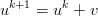## Monday, March 16, 2009

### Complex Step Jacobian-Free Newton-Krylov

Newton's method are really useful for solving non-linear systems.Each iteration of the Newton's method requires solving a linear equation:where the Jacobian is the matrix of partial derivativesand then applying the update to the solution vector:For small systems it is tractable to analytically calculate the Jacobian and invert it directly. This rapidly becomes intractable for realistic engineering problems because the number of unknowns can be on the order of 1e6 (if you have a good workstation) to 1e9 (if you have a big cluster). Rather than explicitly creating a 1e6-squared matrix and inverting it at every iteration, an iterative method that only requires the action of the matrix on a vector can be used (such as conjugate gradient or GMRES). Then we can approximate this matrix vector product bywhich is a good improvement in storage over the matrix approach, but we can improve it still further by using a complex stepThis means we only need one system evaluation to approximate the Jacobian, and much more accurate estimates can be made. As shown in a previous post it is second order accurate, and not as limited by subtractive cancellation as the standard difference approach is.

Here's a simple example of the idea in Maxima

/* trying out complex step finite differences for jacobian free newton
krylov methods */

/* define a simple system of equations */
F : zeromatrix(2,1);
F[1,1] : x*x + 2*x;
F[2,1] : 2*x + x*x;

/* analytic Jacobian */
J : zeromatrix(2,2);
J[1,1] : diff(F[1,1],x);
J[1,2] : diff(F[1,1],x);
J[2,1] : diff(F[2,1],x);
J[2,2] : diff(F[2,1],x);

u : zeromatrix(2,1);
v : zeromatrix(2,1);
u[1,1] : 1.5;
u[2,1] : 1.0;
v[1,1] : 0.2;
v[2,1] : 0.1;

c_step : 0.0 + 1.0e-200*%i; /* complex step */

/* approximate Jacobian using complex step */
Jv_approx : (
ev(F, x = u[1,1] + c_step*v[1,1], x = u[2,1] + c_step*v[2,1])
) / imagpart(c_step);
imagpart(Jv_approx);

/* evaluate the analytic Jacobian to compare */
Jv_analyt : ev(J.v,x=u[1,1],x=u[2,1]);

A good survey article introducing the JFNK method and describing some applications:
Jacobian-free Newton-Krylov methods: a survey of approaches and applications

Nice short paper about using complex steps in finite differences:
Using Complex Variables to Estimate Derivatives of Real Functions